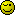# Error on pagination with search results!

I’ve got a mysql database with a table having 3 columns “fname,lname & phone” I have created a search script which perfectly works but what I want is display only 2 data as in 2 rows per page from the ‘users’ table in the respected order! I’ve got around 8 rows, so there should be 4 pages with next & prev buttons!

I’ve then created a pagination code, which shows one row extra i.e 2 rows(the other row doesn’t match with my search results but its a data which is directly below or above it in the mysql databse) with my searched results, the pagination worked perfectly when i directly showed mysql data bt is not working perfectly with search! a error shows up at the top indicating line 16(marked in code)

here is my html form

``````<html>
<title>Search the Database</title>

<body>

<form action="search4.php" method="post">
Search: <input type="text" name="term" /><br />
<input type="submit" name="submit" value="Submit" />
</form>

</body>
</html>
``````

here is my pagination code!

``````<?php

mysql_connect ("localhost", "root","")  or die (mysql_error());
mysql_select_db ("mydata");

\$term = \$_POST['term'];

\$per_page= 2;

\$start= \$_GET['start']; // = 2 <----------------------------------------------line 16

//count record
\$record_count = mysql_num_rows(mysql_query("SELECT * FROM users where fname like '%\$term%'"));

//count max pages
\$max_pages = \$record_count / \$per_page;

if (!\$start)
\$start = 0;

//display data
\$get = mysql_query("SELECT * FROM users LIMIT \$start, \$per_page");
while (\$row = mysql_fetch_assoc(\$get))
{
//get data
\$fname = \$row['fname'];
\$lname = \$row['lname'];
\$phone = \$row['phone'];

echo \$fname." (".\$lname.") (".\$phone.")  <br />";

}

\$prev = \$start- \$per_page;
\$next = \$start+ \$per_page;

if (!(\$start<=0))
echo "<a href='search5.php?start=\$prev'>Prev</a>";

//page numbers

//st var for 1st page
\$i=1;
for (\$x=0; \$x<\$record_count; \$x=\$x+\$per_page)
{
if (\$start!=\$x)

echo "<a href='search5.php?start=\$x'>\$i</a>";
else
echo "<a href='search5.php?start=\$x'><b>\$i</b></a>";
\$i++;

}

//next
if (!(\$start>=\$record_count-\$per_page))
echo "<a href='search5.php?start=\$next'>Next</a>";

?>

``````

You did not show the error message, which is not helpful, but I guess you are trying to access an unset array key (\$_GET[‘start’]).

The answer is simple, either set it with a value you expect (0?)

``````
<form action="search4.php?start=0" method="post">

``````

OR test for its existence before trying to access it:

``````

\$per_page= 2;  // default per page
\$start = 0 ; // default start

if( isset(\$_GET['start']) ) \$start= \$_GET['start']; // override it only if it is set

// now carry on

``````

The latter method might be preferable, it reduces the url cruft and permits you to link to your search from other places and you are able to forget adding the ?start=0 argument. To err is human